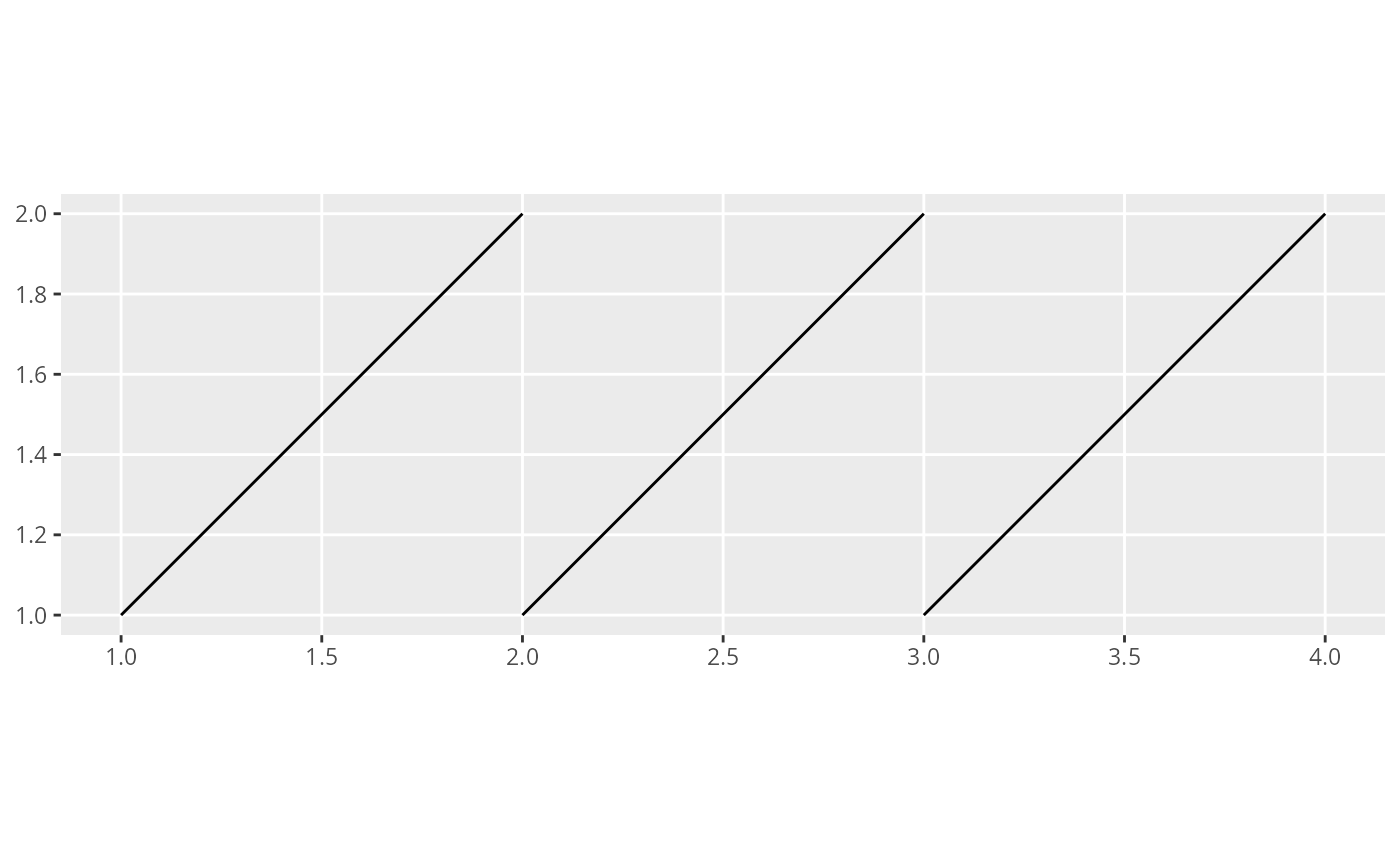A line in 2D space is defined by a start and an and point, each associated with 2D coordinates. This function takes a /codedata.frame as input and assumes that each row defines a line in space. In order to do so, the data frame must have at least four columns and the start.cols and end.cols parameters must be used to point out the names of the columns that define the start and end coordinates of the line. The data is then converted to a SpatialLinesDataFrame DF. If a coordinate reference system crs is provided it is attached to DF. If also to.crs is provided, the coordinate system of DF is transfromed accordingly. Additional columns of the input data, e.g. covariates, are retained and attached to DF.

## Usage

sline(data, start.cols, end.cols, crs = CRS(as.character(NA)), to.crs = NULL)

## Arguments

data

A data.frame

start.cols

Character array poitning out the columns of data that hold the start points of the lines

end.cols

Character array poitning out the columns of data that hold the end points of the lines

crs

Coordinate reference system of the original data

to.crs

Coordinate reference system for the SpatialLines ouput.

## Value

SpatialLinesDataFrame

## Examples

# \donttest{
# Create a data frame defining three lines
lns <- data.frame(
xs = c(1, 2, 3), ys = c(1, 1, 1), # start points
xe = c(2, 3, 4), ye = c(2, 2, 2)
) # end points

# Conversion to SpatialLinesDataFrame without CRS
spl <- sline(lns,
start.cols = c("xs", "ys"),
end.cols = c("xe", "ye")
)

if (require(ggplot2, quietly = TRUE)) {
# Plot the lines
ggplot() +
gg(spl)
}# }